GNSS Geodesy Innovation Interviews UAV/UAS Positioning Surveying Applications Perspective Time Navigation Mapping Policy Land Administration News Archives LBS GIS SDI Imaging E-Zine# A novel approach to autonomous pedestrian navigation

Sep 2012 | No Comment

###### This approach is an alternative to foot-mounted pedestrian navigation systems. A kinetic model of human gait is used as a virtual velocity sensor to curb the divergence of INS computed horizontal velocity and tilt errors## Pavel Davidson

###### Tampere University of Technology, Finland

High-performance autonomous pedestrian dead-reckoning (PDR) systems usually include 6 degrees-offreedom (DOF) inertial measurements unit (IMU) to calculate position of the user. These systems don’t rely on GPS signals and preinstalled infrastructure such as RF beacons, Wi-Fi routers, ultrasonic transmitters etc. Standard inertial navigation system (INS) calculates position by temporal integration of IMU data that comes from three accelerometers and three gyroscopes. Estimated position is calculated at regular time intervals. The unaided INS’s position, velocity and attitude errors grow with time and can be quite significant especially when IMU consists of low-cost MEMS sensors. Therefore traditional unaided INS mechanization is impractical for pedestrian navigation.

One way to curb the divergence of errors in INS is to use external velocity and position aiding. In indoor scenarios without preinstalled infrastructure the options for external velocity and position aiding are very limited: building floor plans can be used for position update, Doppler radars for velocity update. But even these options are not always available.

An alternative navigation method takes advantage of biomechanics of walking. Recognizing that people move one step at a time, the pedestrian mechanization restricts error growth by propagating position estimates in a stride-wise fashion, rather than on a fixed time interval. Inertial sensors in a pedestrian dead reckoning (PDR) system are used to detect the occurrence of steps, and provide a means of estimating the distance and direction in which the step was taken. In this way position error is proportional to the number of steps or traveled distance. Many current PDR systems use foot mounted IMU which allows for zero-velocity update at every stride.

In this paper we propose a novel approach for velocity update based on knowledge of human walking process. It can be applied to PDR systems that include body-mounted IMU at waist or torso, which in some applications can be advantageous compare to foot-mounted sensors. The knowledge of human gait dynamics gives us an alternative way to calculate traveled distance and velocity averaged during the step, which can be considered as a virtual measurement. The different characteristics of errors in INS output and in this virtual measurement make it possible to apply complementary filter methodology and significantly improve INS performance by keeping the horizontal velocity and tilt errors small. The processing of corrected IMU output results in accurate estimation of stride length and direction. This paper presents the real-world results from pedestrian indoor walking tests.

## Dynamics of human gait

Dynamics of human gait is a unique source of external velocity aiding for pedestrian navigation systems that has largely been overlooked by the engineers. Analysis of the human gait shows that the basic pattern of human motion during a walk is cyclical, repeatable and remarkably consistent between individuals . At normal constant walking speed, the vertical and horizontal components of the body’s centre of gravity velocity oscillate smoothly with the frequency equal to the step frequency.

The amplitude of vertical acceleration and vertical displacement are proportional to the step length. As a result, the stride events as well as stride length can be estimated using models of gait dynamics and measured motion parameters. When a foot hits the ground significant vertical acceleration is generated by the impact. Therefore the algorithms for stride occurrence detection can be based on the analysis of the acceleration pattern during walking. For example, the body-mounted accelerometers can detect maximum or minimum of the vertical acceleration, which corresponds to the step occurrences. If the vertical acceleration exceeds the prede fined threshold then the step occurrence is detected. Since the pattern of acceleration depends on type of movement (walking, running, going up or down stairs, etc.) and type of ground over which the person walks (hard, soft, slippery surface) the determination of threshold for reliable step detection is not so easy .

Another way to process accelerometer data for step detection is to use zerocrossing of the acceleration magnitude signal. The zero-crossing method requires a tri-axial accelerometer to measure pedestrian movement. The magnitude of the acceleration vector can be computed by subtracting the local gravity from the measured magnitude of the acceleration vector. Footfall recognition is based on determination of the zero crossing of the acceleration magnitude signal. Since the norm of the acceleration vector is used for the orientation of the sensor unit, it has no effect on the measurement. Step detection is triggered when zero crossings fit in the recognition window .

The step length depends on several factors such as walking velocity, step frequency and height of walker etc. The algorithms for step length estimation generally fall into two groups: (1) algorithms based on biomechanical models and (2) algorithms based on empirical relationships. The example of the step length estimator based on empirical relation between the vertical acceleration and the step length is given by

where amax, amin are the maximum and minimum values of the vertical acceleration during the step. These algorithms show good performance during normal walking on flat terrain. The step length estimation error is 2-5% of distance . The performance of this algorithm degrades rapidly when a person is walking, for example, on non-flat terrain or climbing on stairs.

Average speed during the step can be calculated as the ratio between the step length and step duration. This ground speed can be also resolved into the north and the east velocity components using INS computed heading averaged over the same step. Thus the estimation of pedestrian velocity during the step is obtained. Since the velocity error of this kinetic model based estimator is a result of several independent error sources such as model error, measurement error, inaccuracy in step occurrence events it has a random distribution and can be approximated as Gaussian noise.

## Fusion of IMU data and pedestrian dynamics

In our pedestrian navigation system, the body mounted IMU contains three gyros and three accelerometers that measure the projections of absolute angular rate and specific force on their sensitivity axes. The navigation computations are performed in the local-level coordinate frame. The transformation matrix from the sensor frame to the local-level frame is calculated using output from gyroscopes. The mechanization equations are implemented as local-level terrestrial navigator without vertical channel.

Position, velocity and attitude errors in a stand-alone INS grow with time. For short period of autonomous operation (1-1.5 hours) the propagation of horizontal velocity errors and tilts can be approximated by the Schuler oscillations. When the INS is assumed to be nominally level with altitude compensation and constant low speed, the single north channel error model is described by the following equations :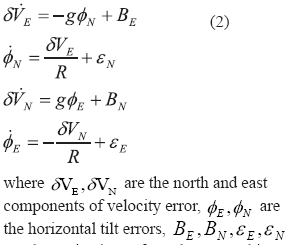are the projections of accelerometer biases and gyro drifts on the local-level frame. The equations for the east channel are similar to eqs. 2. Note that under above assumptions the north, east and vertical channels are not coupled and can be processed separately. The block diagram representation of INS’s north channel is shown in fig. 1 by solid lines 
A well known in control theory approach for oscillation damping is based on feedback loop to control the dynamic behavior of the system. A partially measured output is fed back to the controller where the difference between the reference and the output is amplified to change the input in desired way and obtain improved system performance. The damping of INS errors can be implemented using the external velocity information. The control inputs are shown in fig. 1 by the dashed lines. The gains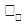andcan be pre-computed or calculated online.

In the case of pedestrian navigation, the kinetic model of gait is considered as a virtual sensor that can be used to estimate the step events and step size for a person while walking. As a result, the estimations of step size and step duration can be used to calculate average velocity over each step. The error of calculated average velocity consists of the errors in step length model, inaccuracy in determining step duration, accelerometer measurement errors. The error components are not correlated with each other thus making the error in velocity random with little correlation in time. Since the error of this estimated average velocity is also not correlated with INS velocity error it can be used as an external velocity measurement for INS.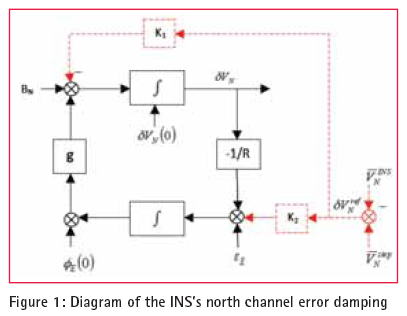Even when a person is walking with almost constant speed, the velocity profile within each step reveals variations of about 0.3 m/sec around the average walking speed. Since the indicated INS velocity follows the same profile, the comparison of momentarily INS velocity with average velocity in not appropriate. Instead, the indicated INS velocity averaged over one step can be compared with average velocity from an external measurement. As a result of this comparison, an estimation of the INS velocity error can be obtained. Since the INS velocity error changes slowly it can be assumed constant during one step. In this case, averaged over one step, INS indicated velocity is calculated as the true average velocity plus the velocity error. The measurement of the INS velocity error can be obtained by taking the difference between INS velocity, averaged over one step and the kinetic model computed velocity: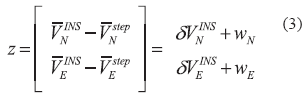Here wN, wE are the velocity errors of the kinetic model based velocity estimator. While calculating the INS velocity error using (3), it was also assumed that the velocity estimated based on the kinetic model has a random error which is assumed to be distributed as a random Gaussian noise. This virtual measurement of INS velocity error can be performed at every step. The mathematical system model for horizontal velocities and tilts is formulated in terms of error state space. Only the north channel equations are described since the data processing for the east channel is similar. Since in pedestrian navigation systems the accelerometer bias has smaller effect than the other error sources there will be three variables of primary interest for each channel: error in INS indicated velocity, tilt error, and gyro drift rate. In terms of these variables, the state differential equations for the north channels become: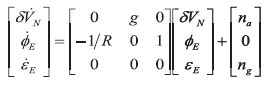where na, ng, is the noise. The measurement to be used as the input to the Kalman filter is the difference between average velocities given by eqs. 3 .

## Experimental results

The proposed method for INS velocity aiding was tested with actual data from the indoor walking tests. The 170 meter test route inside the typical office building is shown in fig. 3. The true pedestrian path is shown by green solid line. The test scenario included different types of movement: walking with variable step length and frequency, going up or down stairs, standing, making sharp turns and opening the doors. The total time of the test was about 5 minutes. The pedestrian dead-reckoning system included 6 DOF IMU, which is composed of three VTI Technologies SCC1300-D02 combined gyroscope and accelerometer with digital SPI interfaces . This is a self-contained device for inertial data collection. In addition to three gyros and accelerometers, it includes also the batteries, memory card and all the necessary electronics for data collection. The complete unit is shown in fig. 2.

The INS computed path is shown in fig. 3 by the magenta asterisks. Our method can reduce only the distance error in PDR systems. Along-track (distance) error during this test does not exceed 2% of travelled distance. The results can be improved when horizontal gyros’ drifts are compensated. But the largest error is caused by heading error. Heading cannot be corrected with this algorithm and other methods, for example, map-matching can be used to reduce the heading error.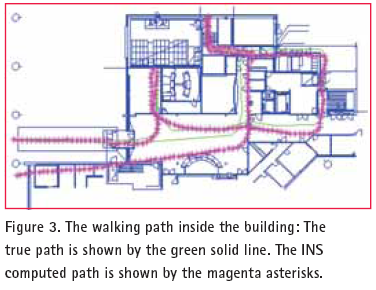## Conclusions

We proposed a novel approach for INS external velocity aiding in pedestrian navigation systems. Our approach can be applied to body-mounted INS which in some applications can be advantageous compare to foot-mounted sensors mainly because the body mounted IMU is isolated from shocks and high dynamics. For the same MEMS gyros the measurement error can be significantly higher if the gyros are exposed to shocks. Therefore the PDR systems with body mounted IMU have better performance in heading estimation. In addition to this, the body mounted IMU is preferable for the motion classification.

The method uses kinetic model of human gait which gives us an alternative way to calculate traveled distance and velocity averaged during the step which can be considered as a virtual velocity measurement. The different characteristics of errors in INS output and in this virtual measurement make it possible to apply complementary filter methodology and significantly improve INS performance by keeping the horizontal velocity and tilt errors small. The distance error is about 2% of traveled distance. Our approach does not have restrictions on pedestrian movement. However reliable velocity update is possible only during normal walking or standing.

## References

 Stirli ng R., “Development of a Pedestrian Navigation System Using Shoe Mounted Sensors,” MSc thesis, University of Alberta, 2004.

 Ladetto Q., “On foot navigation: continuous step calibration using both complementary recursive prediction and adaptive Kalman filtering,” in proceedings of ION GPS conference, 2000.

 Ladetto, Q., Merminod B., “Digital Magnetic Compass and Gyroscope Integration for Pedestrian Navigation” 9th International Conference on Integrated Navigation Systems, St- Petersburg, May 27-29, 2002

 Jahn J ., Batzer U., Seitz J., Patino- Studencka L. and Boronat J., “Comparison and Evaluation of Acceleration Based Step Length Estimators for Handheld Devices,” International Conference on Indoor Positioning and Indoor Navigation, Zürich, Switzerland, 15-17 September 2010.

 Matthews C., Ketema Y., Gebre- Egziabher D., Schwartz M., “In- Situ Step Size Estimation Using a Kinetic Model of Human Gait,” in proceedings of ION GNSS, Portland, OR, September 21-24, 2010.

 Ashkenazy Y., Hausdorf J., Ivanov P., Stanley E., “A stochastic model of human gait dynamics”, Physica A: Statistical Mechanics and its applications, vol. 316, issue 1-4 December 2002.

 Yan Cui, Ariyur, K.B., “Bounding inertial drift with human gait dynamics for personal navigation”, IEEE International Systems conference, 2011.

 Farrell J., and Barth M. 1999. “The Global Positioning System and Inertial Navigation,” McGraw-Hill, 1999

 Salychev O., “Applied Inertial Navigation: Problems and Solutions,” BMSTU Press. Moscow. 2004

 David son P., and Takala J., “Algorithm for Pedestrian Navigation Combining IMU Measurements and Gait Models,” 19th International Conference on Integrated Navigation Systems, St- Petersburg, May 28-30, 2012

 VTI Technologies Oy, “SCC1300 gyroscope” datasheet, http:// www.vti.fi/en

##### EDITORIAL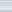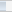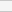(2 votes, average: 2.50 out of 5)Loading...